# MySQL: size of your tables – tricks and tips

on

Many of you already know how to retrieve the size of your dataset, schemas and tables in MySQL.

To summarize, below are the different queries you can run:

## Dataset Size

I the past I was using something like this :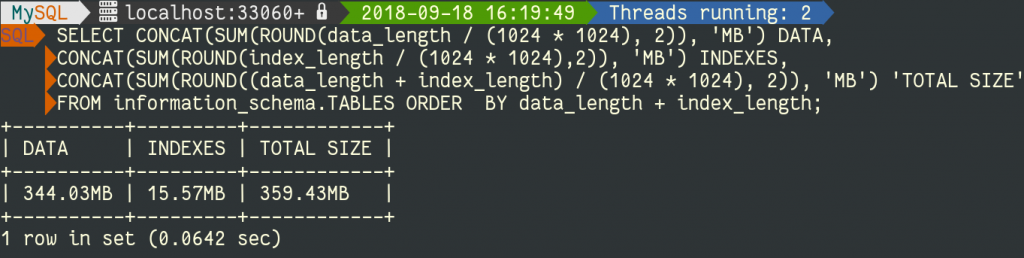But now with `sys schema` being installed by default, I encourage you to use some of the formatting functions provided with it. The query to calculate the dataset is now:

```SELECT sys.format_bytes(sum(data_length)) DATA,
sys.format_bytes(sum(index_length)) INDEXES,
sys.format_bytes(sum(data_length + index_length)) 'TOTAL SIZE'
FROM information_schema.TABLES ORDER  BY data_length + index_length;
```

## Engines Used and Size

For a list of all engines used:

```SELECT count(*) as '# TABLES', sys.format_bytes(sum(data_length)) DATA,
sys.format_bytes(sum(index_length)) INDEXES,
sys.format_bytes(sum(data_length + index_length)) 'TOTAL SIZE',
engine `ENGINE` FROM information_schema.TABLES
WHERE TABLE_SCHEMA NOT IN ('sys','mysql', 'information_schema',
GROUP BY engine;
```

Let’s see an example on the same database as above: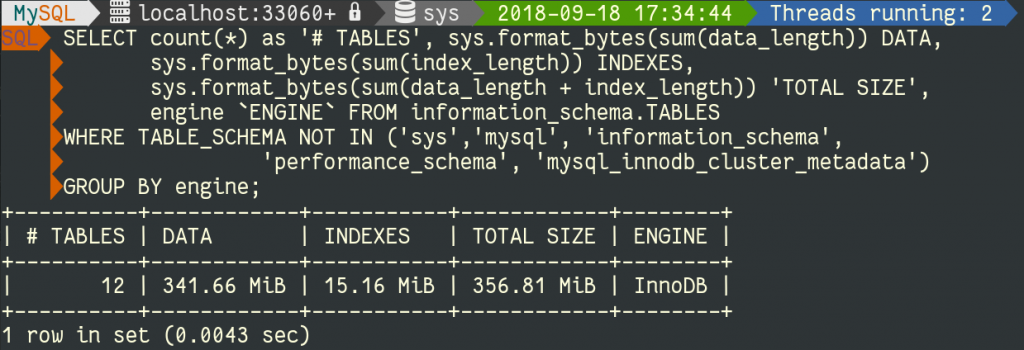and on 5.7 with one MyISAM table (eeek):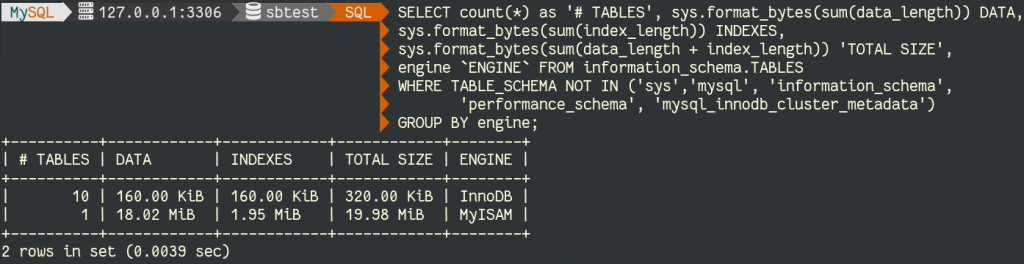## Schemas Size

Now let’s find out which schemas are the larges:

```SELECT TABLE_SCHEMA,
sys.format_bytes(sum(table_rows)) `ROWS`,
sys.format_bytes(sum(data_length)) DATA,
sys.format_bytes(sum(index_length)) IDX,
sys.format_bytes(sum(data_length) + sum(index_length)) 'TOTAL SIZE',
round(sum(index_length) / sum(data_length),2) IDXFRAC
FROM
information_schema.TABLES
GROUP By table_schema ORDER BY sum(DATA_length) DESC;
```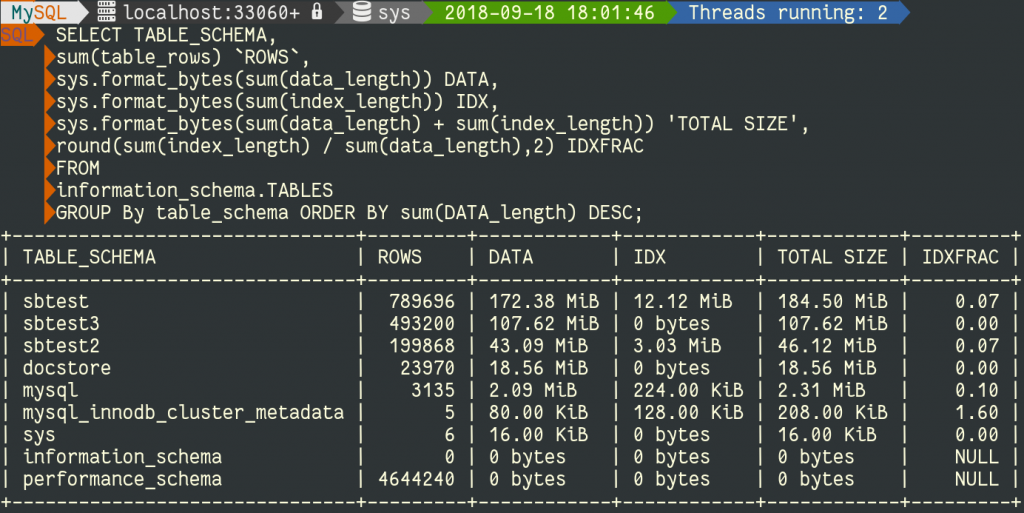## Top 10 Tables by Size

And finally a query to get the list of the 10 largest tables:

```SELECT CONCAT(table_schema, '.', table_name) as 'TABLE', ENGINE,
CONCAT(ROUND(table_rows / 1000000, 2), 'M')  `ROWS`,
sys.format_bytes(data_length) DATA,
sys.format_bytes(index_length) IDX,
sys.format_bytes(data_length + index_length) 'TOTAL SIZE',
round(index_length / data_length,2) IDXFRAC
FROM   information_schema.TABLES
ORDER  BY data_length + index_length DESC LIMIT  10;
```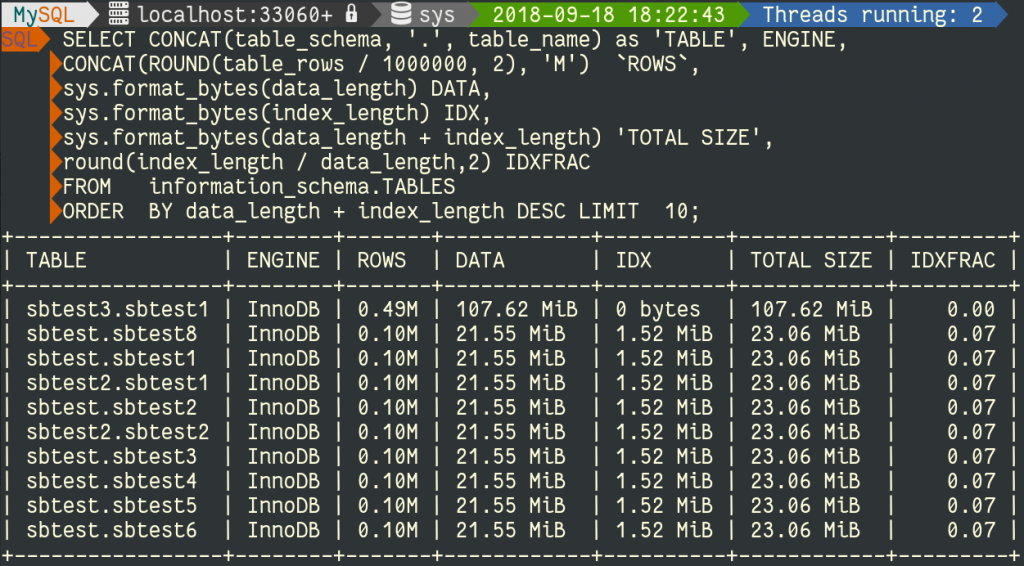You can modify the query to retrieve the size of any given table of course.

That was the theory and it’s always good to see those queries time to time.

## But…

But can we trust these results ? In fact, sometimes, this can be very tricky, let’s check this example:

```mysql> SELECT COUNT(*) AS TotalTableCount ,table_schema,
CONCAT(ROUND(SUM(table_rows)/1000000,2),'M') AS TotalRowCount,
CONCAT(ROUND(SUM(data_length)/(1024*1024*1024),2),'G') AS TotalTableSize,
CONCAT(ROUND(SUM(index_length)/(1024*1024*1024),2),'G') AS TotalTableIndex,
CONCAT(ROUND(SUM(data_length+index_length)/(1024*1024*1024),2),'G') TotalSize
FROM information_schema.TABLES GROUP BY table_schema
ORDER BY SUM(data_length+index_length)  DESC LIMIT 1;
+-----------------+--------------+---------------+----------------+-----------------+-----------+
| TotalTableCount | TABLE_SCHEMA | TotalRowCount | TotalTableSize | TotalTableIndex | TotalSize |
+-----------------+--------------+---------------+----------------+-----------------+-----------+
|              15 | wp_lefred    | 0.02M         | 5.41G          | 0.00G           | 5.41G     |
+-----------------+--------------+---------------+----------------+-----------------+-----------+
```

This seems to be a very large table ! Let’s verify this:

```mysql> select * from information_schema.TABLES
where table_schema='wp_lefred' and table_name = 'wp_options'\G
*************************** 1. row ***************************
TABLE_CATALOG: def
TABLE_SCHEMA: wp_lefred
TABLE_NAME: wp_options
TABLE_TYPE: BASE TABLE
ENGINE: InnoDB
VERSION: 10
ROW_FORMAT: Dynamic
TABLE_ROWS: 3398
AVG_ROW_LENGTH: 1701997
DATA_LENGTH: 5783388160
MAX_DATA_LENGTH: 0
INDEX_LENGTH: 442368
DATA_FREE: 5242880
AUTO_INCREMENT: 1763952
CREATE_TIME: 2018-09-18 00:29:16
UPDATE_TIME: 2018-09-17 23:44:40
CHECK_TIME: NULL
TABLE_COLLATION: utf8mb4_unicode_ci
CHECKSUM: NULL
CREATE_OPTIONS:
TABLE_COMMENT:
1 row in set (0.01 sec)
```

In fact we can see that the average row length is pretty big.So let’s verify on the disk:

```[root@vps21575 database]# ls -lh wp_lefred/wp_options.ibd
-rw-r----- 1 mysql mysql 11M Sep 18 00:31 wp_lefred/wp_options.ibd
```

11M ?! The table is 11M but `Information_Schema` thinks it’s 5.41G ! Quite a big difference !

In fact this is because InnoDB creates these statistics from a very small amount of pages by default.
So if you have a lot of records with a very variable size like it’s the case with this WordPress table, it could be safer to
increase the amount of pages used to generate those statistics:

```mysql> set global innodb_stats_transient_sample_pages= 100;
Query OK, 0 rows affected (0.00 sec)

mysql> analyze table wp_lefred.wp_options;
+----------------------+---------+----------+----------+
| Table                | Op      | Msg_type | Msg_text |
+----------------------+---------+----------+----------+
| wp_lefred.wp_options | analyze | status   | OK       |
+----------------------+---------+----------+----------+
1 row in set (0.05 sec)

Let's check the table statistics now:```
```*************************** 1. row ***************************
TABLE_CATALOG: def
TABLE_SCHEMA: wp_lefred
TABLE_NAME: wp_options
TABLE_TYPE: BASE TABLE
ENGINE: InnoDB
VERSION: 10
ROW_FORMAT: Dynamic
TABLE_ROWS: 3075
AVG_ROW_LENGTH: 1198
DATA_LENGTH: 3686400
MAX_DATA_LENGTH: 0
INDEX_LENGTH: 360448
DATA_FREE: 4194304
AUTO_INCREMENT: 1764098
CREATE_TIME: 2018-09-18 00:34:07
UPDATE_TIME: 2018-09-18 00:32:55
CHECK_TIME: NULL
TABLE_COLLATION: utf8mb4_unicode_ci
CHECKSUM: NULL
CREATE_OPTIONS:
TABLE_COMMENT:
1 row in set (0.01 sec)
```

We can see that the average row length is much smaller now (and could be smaller with an even bigger sample).

Let’s verify:

```mysql> SELECT CONCAT(table_schema, '.', table_name) as 'TABLE', ENGINE,
-> CONCAT(ROUND(table_rows / 1000000, 2), 'M')  `ROWS`,
-> sys.format_bytes(data_length) DATA,
-> sys.format_bytes(index_length) IDX,
-> sys.format_bytes(data_length + index_length) 'TOTAL SIZE',
-> round(index_length / data_length,2) IDXFRAC
-> FROM   information_schema.TABLES
-> WHERE table_schema='wp_lefred' and table_name = 'wp_options';
+----------------------+--------+-------+----------+------------+------------+---------+
| TABLE                | ENGINE | ROWS  | DATA     | IDX        | TOTAL SIZE | IDXFRAC |
+----------------------+--------+-------+----------+------------+------------+---------+
| wp_lefred.wp_options | InnoDB | 0.00M | 3.52 MiB | 352.00 KiB | 3.86 MiB   |    0.10 |
+----------------------+--------+-------+----------+------------+------------+---------+
```

In fact, this table uses a long text as column and it can be filled with many things or almost nothing.

We can verify that for this particular table we have some very large values:

```mysql> select CHAR_LENGTH(option_value), count(*)
from wp_lefred.wp_options group by 1 order by 1 desc limit 10;
+---------------------------+----------+
| CHAR_LENGTH(option_value) | count(*) |
+---------------------------+----------+
|                    245613 |        1 |
|                    243545 |        2 |
|                    153482 |        1 |
|                    104060 |        1 |
|                     92871 |        1 |
|                     70468 |        1 |
|                     60890 |        1 |
|                     41116 |        1 |
|                     33619 |        5 |
|                     33015 |        2 |
+---------------------------+----------+
```

Even if the majority of the records are much smaller:

```mysql> select CHAR_LENGTH(option_value), count(*)
from wp_lefred.wp_options group by 1 order by 2 desc limit 10;
+---------------------------+----------+
| CHAR_LENGTH(option_value) | count(*) |
+---------------------------+----------+
|                        10 |     1485 |
|                        45 |      547 |
|                        81 |      170 |
|                         6 |      167 |
|                         1 |       84 |
|                        83 |       75 |
|                        82 |       65 |
|                        84 |       60 |
|                        80 |       44 |
|                        30 |       42 |
+---------------------------+----------+
```

## Conclusion

So in general, using `Information_Schema` provides a good overview of the tables size, but please always verify the size on disk to see if it matches because when a table contains records that can have a large variable size, those statistics are often incorrect because the InnoDB page sample used is too small.

But don’t forget that on disk, table spaces can also be fragmented !

## 9 thoughts on “MySQL: size of your tables – tricks and tips”

1.Brian says:

The reason the table appeared very large at first is because your original query is missing the where condition “WHERE table_schema=’wp_lefred’ and table_name = ‘wp_options'”

1.lefred says:

Thx for your comment Brian, in fact that large table is mostly the only large one in that schema. I limited to 1 because other schemas are not interesting. (you can see it from the top 10 largest table) But good catch if you want to reproduce this on your own database.
Cheers,

1.Brian says:

Thanks for the clarification.

2.sobot says:

Very good article. Any idea on how the statistics are created or calculated for tokuDB engine (on Percona or MariaDB)? Is there an equivalent of the “innodb_stats_transient_sample_pages” variable. I can’t find any information on this and have problems with toku on incorrect statistics in the “INFORMATION_SCHEMA.STATISTICS” and “INFORMATION_SCHEMA.Tables” tables.

1.lefred says:

Hi Sobot,
I’ve no idea about TokuDB, which is a discontinued engine even on Percona Server IIRC.

1.sobot says:

Ok thanks anyhow, and yeah I guess they recommend MyRocks so will look into that.

2.boshi fan says:

Hi lefred,
Are you using mysql cli? the prompt is so cool! could you shared it ?🤤

1.boshi fan says:

uh.. it’s mysql shell.

This site uses Akismet to reduce spam. Learn how your comment data is processed.

recent